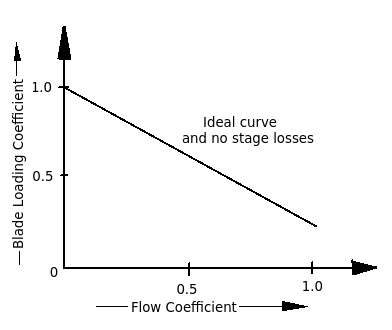Q.  What is the effect of increasing flow coefficient (Φ) in an axial flow compressor on blade loading coefficient (Ψ)?
- Published on 16 Sep 15

d. Unpredictable

#### Discussion

• Pankaj   -Posted on 16 Oct 15
- Flow coefficient, Φ for an axial flow compressor can be defined as the ratio of axial velocity of flow (Cf) to the peripheral velocity of the blades (Cb).

∴ Φ = (Axial velocity of flow Cf) / (Peripheral velocity of the blades Cb)

Ψ = Workdone / (Blade Velocity)2

Ψ = W / (Cb)2

- Blade loading coefficient for an axial flow compressor decreases with increase in the flow coefficient for the same compressor as show in diagram.The performance of 50% reaction axial flow compressor can be predicted by using blade loading coefficient and flow coefficient.

## ➨ Post your comment / Share knowledgeEnter the code shown above:

(Note: If you cannot read the numbers in the above image, reload the page to generate a new one.)